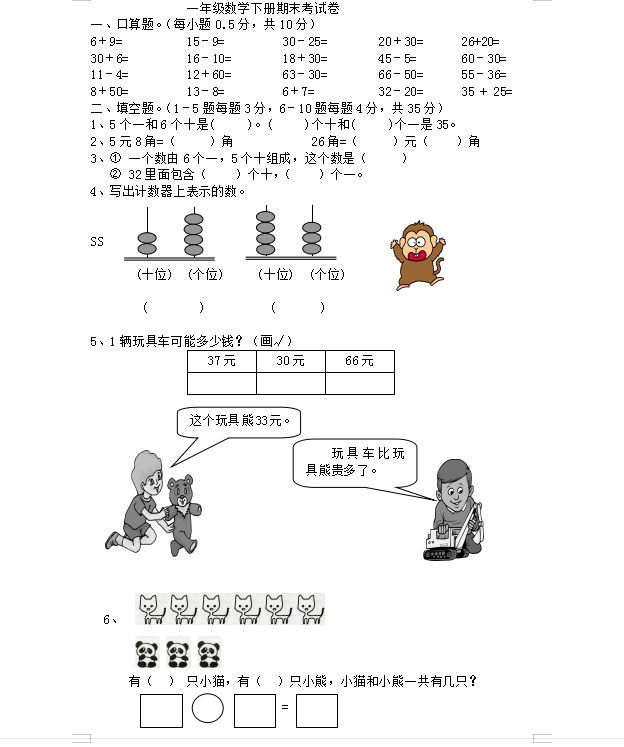6＋9=          15－9=         30－25=        20＋30=      26+20=

30＋6=         16－10=        18＋30=        45－5=       60－30=

11－4=         12＋60=        63－30=        66－50=      55－36=

8＋50=         13－8=         6＋7=          32－20=      35 + 25=